Next: Inverse Functions Up: lab_template Previous: lab_template

# Exponentials and Logarithms

When the exponential function
was introduced, (for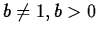) you saw that the function is increasing if
and decreasing if
. You can observe the monotonicity by plotting
and
.
> plot(exp(x),x=-1..1);
> plot(0.1^x,x=-1..1);

The logarithmic functionwas introduced for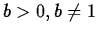as the inverse of the exponential. The logarithm is therefore inreasing ifand decreasing if
. Indeed you can plot
and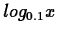.
> plot(log(x),x=0.1..10);
> plot(log[0.1](x),x=0.1..10);

From the monotonicity properties you can compare two logarithms having the same base without computing their values:
We can also see what is the behavior of the logarithm of different bases by using the
command. To get the animation to play, just click on the graph and click on the go button in the tool bar.
> with(plots):
> animate(log[b](x),x=0.1..10,b=1.1..10,frames=30);
> animate(log[b](x),x=0.1..10,b=0.01..0.1,frames=30);

From the monotonicuty properties you can see that for a fixed
in the interval
the logarithm increases withbut forin the interval
it decreases. As a result you can now compare logarithms with different bases without computing their values.
Here are some examples using Maple to solve logarithmic and exponential equations.
> solve(5^(x+1)+5^x+5^(x-1)=155,x);

> solve(log(2*x^2+2*x+5)=2,x);Next: Inverse Functions Up: lab_template Previous: lab_template
Jane E Bouchard
2008-12-03